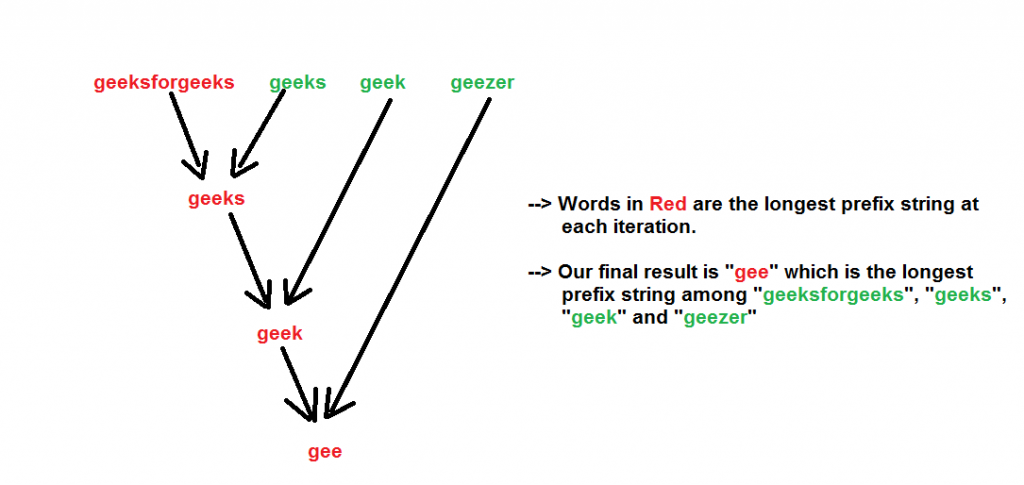GeeksforGeeks App
Open AppBrowser
Continue

# Java Program To Find Longest Common Prefix Using Word By Word Matching

Given a set of strings, find the longest common prefix.
Examples:

```Input  : {“geeksforgeeks”, “geeks”, “geek”, “geezer”}
Output : "gee"

Input  : {"apple", "ape", "april"}
Output : "ap"```

We start with an example. Suppose there are two strings- “geeksforgeeks” and “geeks”. What is the longest common prefix in both of them? It is “geeks”.
Now let us introduce another word, “geek”. So now what is the longest common prefix in these three words ? It is “geek”
We can see that the longest common prefix holds the associative property, i.e-

```LCP(string1, string2, string3)
= LCP (LCP (string1, string2), string3)

Like here

LCP (“geeksforgeeks”, “geeks”, “geek”)
=  LCP (LCP (“geeksforgeeks”, “geeks”), “geek”)
=  LCP (“geeks”, “geek”) = “geek”```

So we can make use of the above associative property to find the LCP of the given strings. We one by one calculate the LCP of each of the given string with the LCP so far. The final result will be our longest common prefix of all the strings.
Note that it is possible that the given strings have no common prefix. This happens when the first character of all the strings are not the same.
We show the algorithm with the input strings- “geeksforgeeks”, “geeks”, “geek”, “geezer” by the below figure.Below is the implementation of above approach:

## Java

 `// Java Program to find the longest``// common prefix``class` `GFG {` `// A Utility Function to find the common``// prefix between strings- str1 and str2``static` `String commonPrefixUtil(String str1,``                               ``String str2)``{``    ``String result = ``""``;``    ``int` `n1 = str1.length(),``        ``n2 = str2.length();` `    ``// Compare str1 and str2``    ``for` `(``int` `i = ``0``, j = ``0``; i <= n1 - ``1` `&&``             ``j <= n2 - ``1``; i++, j++)``    ``{``        ``if` `(str1.charAt(i) != str2.charAt(j))``        ``{``            ``break``;``        ``}``            ``result += str1.charAt(i);``    ``}``        ``return` `(result);``}` `// A Function that returns the longest``// common prefix from the array of strings``static` `String commonPrefix(String arr[],``                           ``int` `n)``{``    ``String prefix = arr[``0``];` `    ``for` `(``int` `i = ``1``; i <= n - ``1``; i++)``    ``{``        ``prefix = commonPrefixUtil(prefix, arr[i]);``    ``}``        ``return` `(prefix);``}` `// Driver code``public` `static` `void` `main(String[] args)``{``    ``String arr[] = {``"geeksforgeeks"``, ``"geeks"``,``                    ``"geek"``, ``"geezer"``};``    ``int` `n = arr.length;``    ``String ans = commonPrefix(arr, n);` `    ``if` `(ans.length() > ``0``)``    ``{``        ``System.out.printf(``"The longest common prefix is - %s"``,``                           ``ans);``    ``}``    ``else``    ``{``        ``System.out.printf(``"There is no common prefix"``);``    ``}``}``}``// This code is contributed by 29AjayKumar`

Output :

`The longest common prefix is - gee`

Time Complexity : Since we are iterating through all the strings and for each string we are iterating though each character, so we can say that the time complexity is O(N M) where,

```N = Number of strings
M = Length of the largest string string ```

Auxiliary Space : To store the longest prefix string we are allocating space which is O(M). Please refer to complete article on Longest Common Prefix using Word by Word Matching for more details!

Another approach to Find Longest Common Prefix Using Word By Word Matching, in this we use divide and conquer approach. The basic idea of this approach is to divide the array of string into smaller subarrays and then find the common prefix among them recursively.it uses the recursive function commonPrefix(arr, low, high) that takes an array of strings. A lower bound, and an upper bound as input. It divides the array into two smaller subarrays recursively and finds the common prefix among them by calling the commonPrefixUtil(str1, str2) function.

## Java

 `public` `class` `LongestCommonPrefix {``    ``// recursive method to find the longest common prefix``    ``static` `String commonPrefix(String[] arr, ``int` `low, ``int` `high) {``        ``// base case: if the array has only one element, return it``        ``if` `(low == high)``            ``return` `(arr[low]);` `        ``// recursive case: divide the array into two sub-arrays``        ``if` `(high > low) {``            ``int` `mid = low + (high - low) / ``2``;` `            ``// find the common prefix of the left sub-array``            ``String str1 = commonPrefix(arr, low, mid);``            ``// find the common prefix of the right sub-array``            ``String str2 = commonPrefix(arr, mid + ``1``, high);` `            ``// compare the two sub-arrays and return the common prefix``            ``return` `commonPrefixUtil(str1, str2);``        ``}``        ``// in case of no common prefix, return an empty string``        ``return` `""``;``    ``}` `    ``// helper method to compare two strings and find their common prefix``    ``static` `String commonPrefixUtil(String str1, String str2) {``        ``String result = ``""``;``        ``int` `n1 = str1.length(),``                ``n2 = str2.length();` `        ``// compare characters at each index of the two strings``        ``for` `(``int` `i = ``0``, j = ``0``; i <= n1 - ``1` `&& j <= n2 - ``1``; i++, j++) {``            ``// if the characters are not the same, return the result``            ``if` `(str1.charAt(i) != str2.charAt(j))``                ``break``;``            ``// otherwise, add the character to the result``            ``result += str1.charAt(i);``        ``}``        ``return` `(result);``    ``}` `    ``public` `static` `void` `main(String[] args) {``        ``// array of strings to find the common prefix``        ``String[] arr = {``"geeksforgeeks"``, ``"geeks"``, ``"geek"``, ``"geezer"``};``        ``int` `n = arr.length;` `        ``// call the commonPrefix method and store the result``        ``String ans = commonPrefix(arr, ``0``, n - ``1``);` `        ``// print the result``        ``if` `(ans.length() > ``0``) {``            ``System.out.printf(``"The longest common prefix is - %s"``, ans);``        ``} ``else` `{``            ``System.out.printf(``"There is no common prefix"``);``        ``}``    ``}``}`

Output

`The longest common prefix is - gee`

Time complexity: O(NM log(N))

Auxiliary space: O(log(N))

My Personal Notes arrow_drop_up